#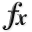N-D Test Functions X¶

class go_benchmark.XinSheYang01(dimensions=2)

Xin-She Yang 1 test objective function.

This class defines the Xin-She Yang 1 global optimization problem. This is a multimodal minimization problem defined as follows: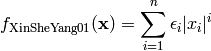The variable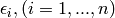is a random variable uniformly distributed in.

Here,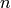represents the number of dimensions andfor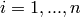.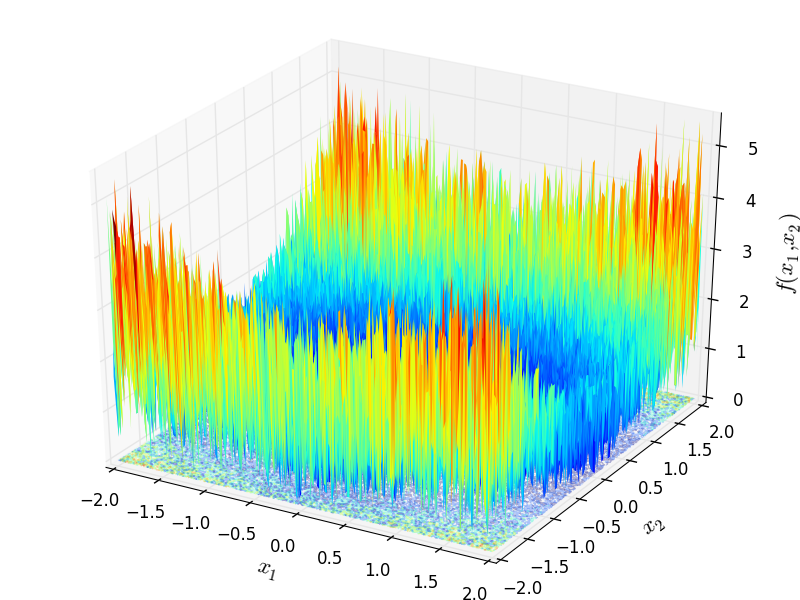Two-dimensional Xin-She Yang 1 function

Global optimum: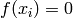for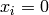forclass go_benchmark.XinSheYang02(dimensions=2)

Xin-She Yang 2 test objective function.

This class defines the Xin-She Yang 2 global optimization problem. This is a multimodal minimization problem defined as follows: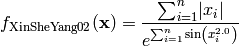Here,represents the number of dimensions and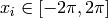for.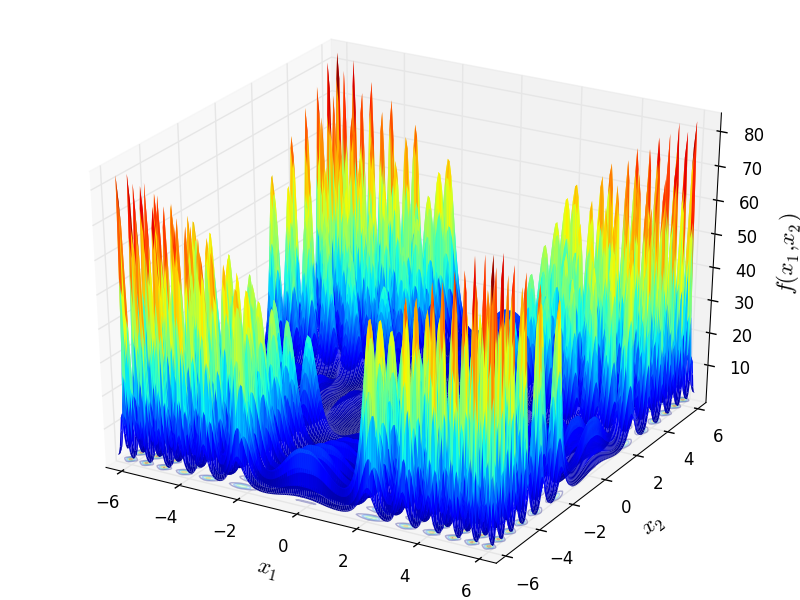Two-dimensional Xin-She Yang 2 function

Global optimum:forforclass go_benchmark.XinSheYang03(dimensions=2)

Xin-She Yang 3 test objective function.

This class defines the Xin-She Yang 3 global optimization problem. This is a multimodal minimization problem defined as follows: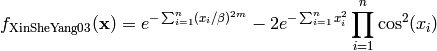Where, in this exercise,and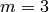.

Here,represents the number of dimensions and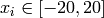for.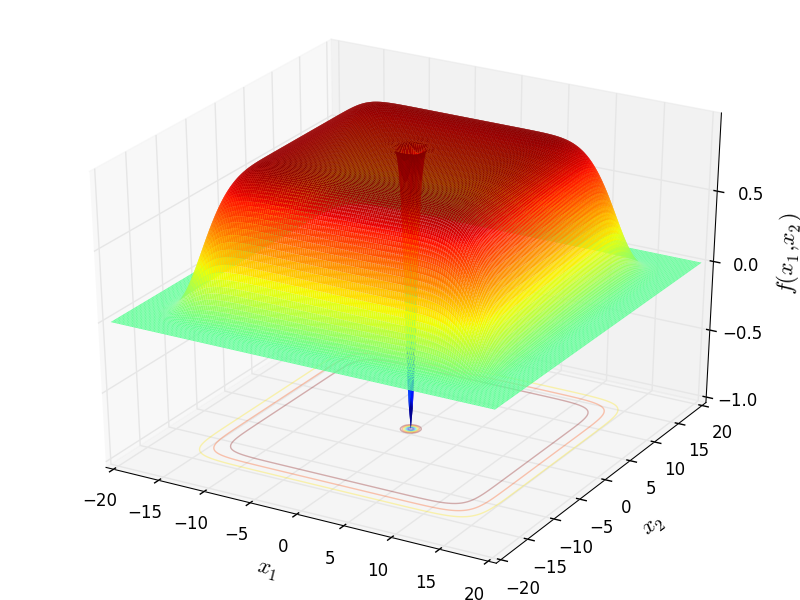Two-dimensional Xin-She Yang 3 function

Global optimum:forforclass go_benchmark.XinSheYang04(dimensions=2)

Xin-She Yang 4 test objective function.

This class defines the Xin-She Yang 4 global optimization problem. This is a multimodal minimization problem defined as follows:Here,represents the number of dimensions andfor.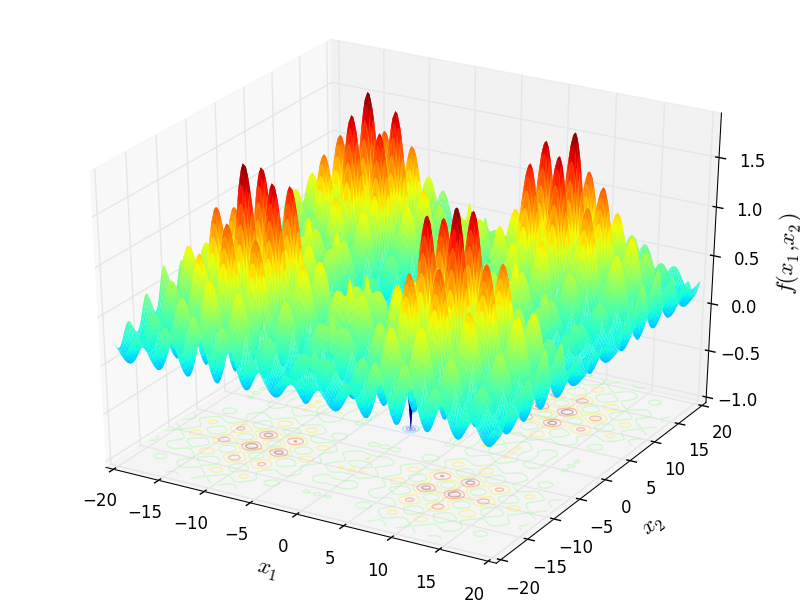Two-dimensional Xin-She Yang 4 function

Global optimum:forforclass go_benchmark.Xor(dimensions=9)

Xor test objective function.

This class defines the Xor global optimization problem. This is a multimodal minimization problem defined as follows: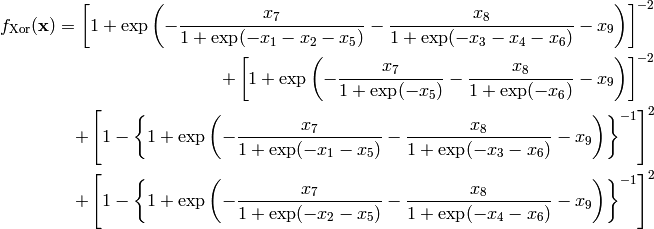Here,represents the number of dimensions and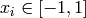for.

Global optimum: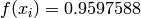for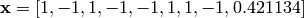#### Previous topic

N-D Test Functions W

#### Next topic

N-D Test Functions Y